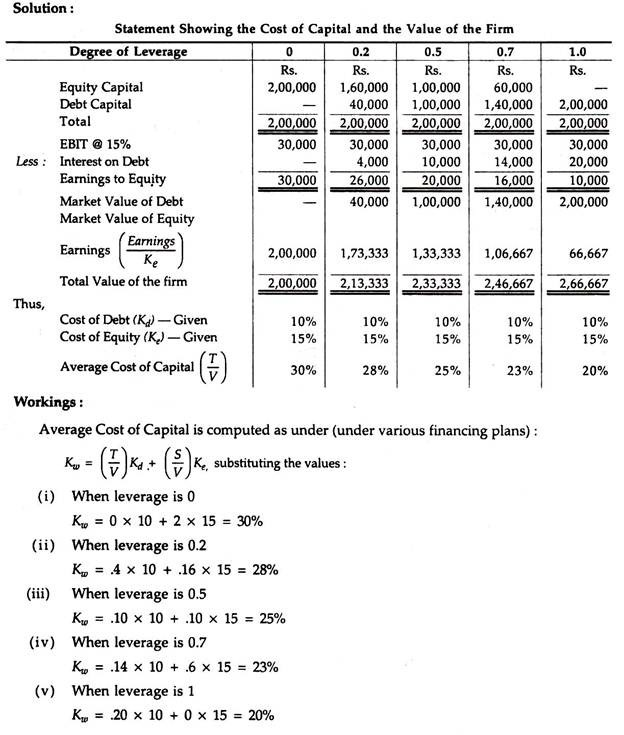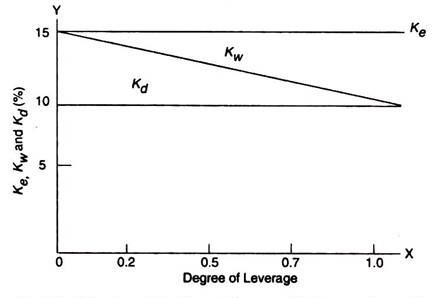# Net Income (NI) Approach

According to NI approach a firm may increase the total value of the firm by lowering its cost of capital.

When cost of capital is lowest and the value of the firm is greatest, we call it the optimum capital structure for the firm and, at this point, the market price per share is maximized.

The same is possible continuously by lowering its cost of capital by the use of debt capital. In other words, using more debt capital with a corresponding reduction in cost of capital, the value of the firm will increase.

The same is possible only when:

(i) Cost of Debt (Kd) is less than Cost of Equity (Ke)

(ii) There are no taxes; and

(iii) The use of debt does not change the risk perception of the investors since the degree of leverage is increased to that extent.

Since the amount of debt in the capital structure increases, weighted average cost of capital decreases which leads to increase the total value of the firm. So, the increased amount of debt with constant amount of cost of equity and cost of debt will highlight the earnings of the shareholders.

Example-

X Ltd. presents the following particulars:

EBIT (i.e., Net Operating income) is Rs. 30,000;

The equity capitalization ratio (i.e., cost of equity) is 15% (Ke);

Cost of debt is 10% (Kd);

Total Capital amounted to Rs. 2,00,000.

Calculate the cost of capital and the value of the firm for each of the following alternative leverage after applying the NI approach.

Leverage (Debt to total Capital) 0%, 20%, 50%, 70% and 100%.From the above table it is quite clear that the value of the firm (V) will be increased if there is a proportionate increase in debt capital but there will be a reduction in overall cost of capital. So, Cost of Capital is increased and the value of the firm is maximum if a firm uses 100% debt capital.

It is interesting to note the NI approach can also be graphically presented as under (with the help of the above example)The degree of leverage is plotted along the X-axis whereas Ke, Kw and Kd are on the Y-axis. It reveals that when the cheaper debt capital in the capital structure is proportionately increased, the weighted average cost of capital, Kw, decreases and consequently the cost of debt is Kd.

Thus, it is needless to say that the optimal capital structure is the minimum cost of capital if financial leverage is one; in other words, the maximum application of debt capital.

The value of the firm (V) will also be the maximum at this point.

## 2 thoughts on “Net Income (NI) Approach”

error: Content is protected !!